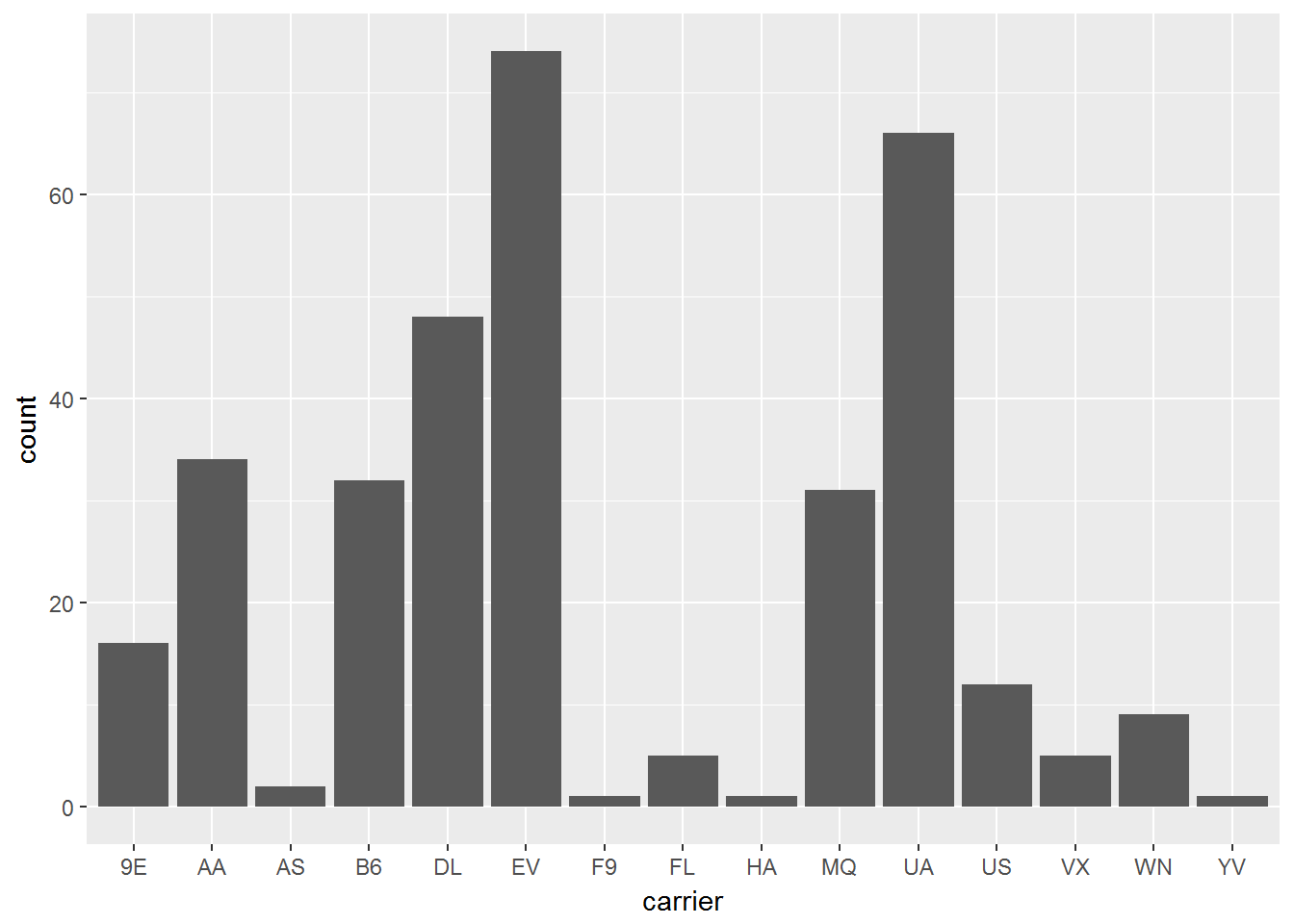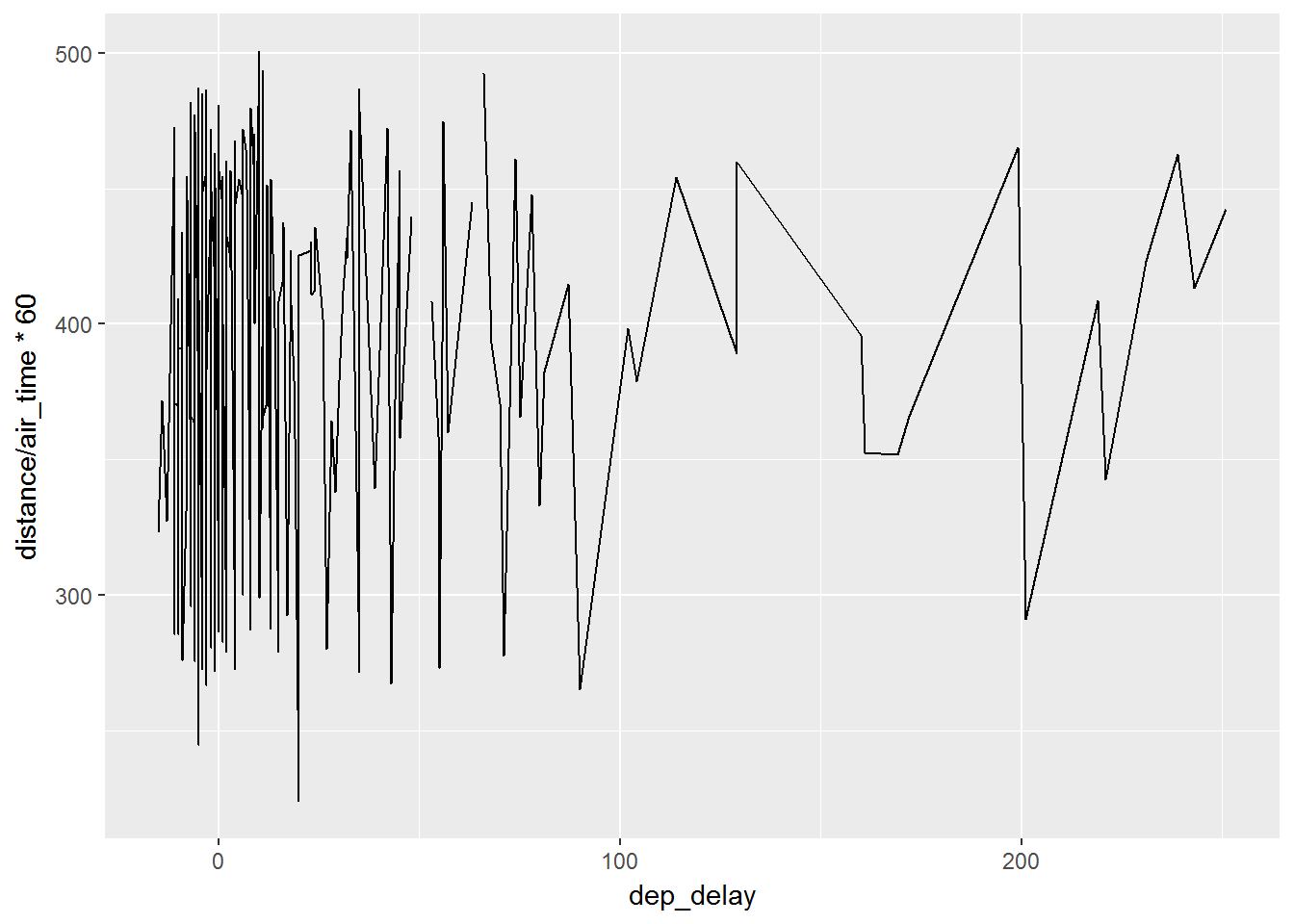# Extra Graphs

We will display the code for a couple for common graphs below:

## Bar Charts

``````ggplot(data, aes( carrier) )+
geom_bar()``````## Line and Path plots

``````ggplot(data, aes(dep_delay, distance/air_time*60 )) +
geom_line()``````